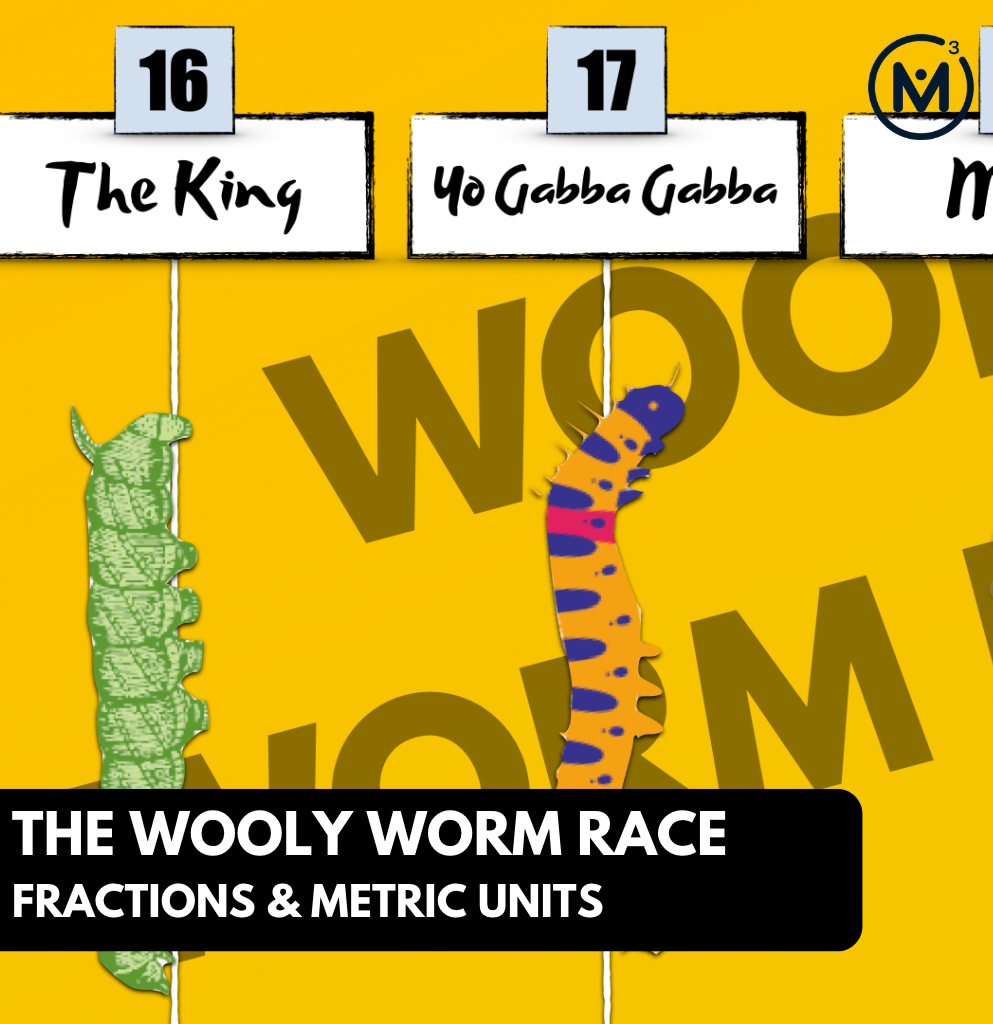## THE WOOLY WORM RACE [DAY 6]

### FRACTIONS & METRIC UNITS

Represent, compare, order, and add fractions involving metric unit conversions.

## Intentionality & Unit Overview### Access each lesson from this unit using the navigation links below

Students will demonstrate the concepts and skills that they have developed through this context and learning experience.

## Intentionality…

The purpose of the Day 6 assessment is for students to demonstrate their understanding of the following big ideas.

Number:

• Fractions can be represented in a variety of ways;
• How you partition the whole determines the fractional unit (i.e.: partitioning a whole into 6 parts will result in 6 sixth parts);
• As you partition a whole into more parts, the smaller the size of each part;
• As you partition a whole into more parts, the larger the denominator;
• The numerator indicates the number of parts relative to the number of parts in the whole indicated by the denominator;
• Fractional amounts exist between whole numbers;
• Different denominators can be used to represent equivalent quantities;
• A multiplicative relationship exists between unit fractions (i.e.: one-sixth is one-half of one-third and one third is twice as big as one-sixth); and,
• The commutative and associative properties of addition (this big idea may emerge through student strategies)
• Quantities represented as a decimal are fractions limited to base ten denominators (i.e.: tenths, hundredths, thousandths, etc.)
• Quantities represented as a percent are fractions where the denominator is 100.

Measurement:

• Units of measure have different sizes and attributes; and,
• As the size of the unit of measure decreases, the number of iterations of that unit required to measure a quantity increases (and vice versa).

The independent assessment will allow students to demonstrate concepts and skills related to the following learning goals:

• I will represent fractions.
• I will order and compare fractions.
• I will represent fractions expressed symbolically in words as well as in standard notation including proper and improper fractions as well as mixed numbers.
• I will add fractions with friendly denominators.
• I will determine an appropriate unit of measure.
• I will explain the relationship between metres and centimetres.
• I will convert between metres and centimetres.

Students might write and/or model their answers independently. Students can also be interviewed by their teacher.

An alternative assessment opportunity should be offered if this tool will not successfully capture what the student knows, understands or can do.

## Math Talk

In today’s math talk, students will work through a string of related problems to determine which caterpillar won in the two caterpillar division.

Round #1:
Taylor, who travelled one and 3 fourths of a metre
or
Tammy, who travelled 170% of a metre

Round #2:
Sammy, who travelled 1.8 of a metre
or
Swirly, who travelled 1 9/10 of a metre

Login/Join to access the entire Teacher Guide, downloadable slide decks and printable handouts for this lesson and all problem based units.

## Assessment Considerations…

Login/Join to access the entire Teacher Guide, downloadable slide decks and printable handouts for this lesson and all problem based units.

## Assessment: Representing, Ordering and Adding Fractions & Selecting and Converting Metric Units

Question #1:

Login/Join to access the entire Teacher Guide, downloadable slide decks and printable handouts for this lesson and all problem based units.

Question #2:

Login/Join to access the entire Teacher Guide, downloadable slide decks and printable handouts for this lesson and all problem based units.

Question #3:

Login/Join to access the entire Teacher Guide, downloadable slide decks and printable handouts for this lesson and all problem based units.

Question #4:

Login/Join to access the entire Teacher Guide, downloadable slide decks and printable handouts for this lesson and all problem based units.

## Considerations to Promote Student Growth

Login/Join to access the entire Teacher Guide, downloadable slide decks and printable handouts for this lesson and all problem based units.

Login/Join to access the entire Teacher Guide, downloadable slide decks and printable handouts for this lesson and all problem based units.

## Educator Discussion Area

Login/Join to access the entire Teacher Guide, downloadable slide decks and printable handouts for this lesson and all problem based units.

## Explore Our 60+ Problem Based Units

This Make Math Moments Lesson was designed to spark curiosity for a multi-day unit of study with built in purposeful practice, number talks and extensions to elicit and emerge strategies and mathematical models.

Dig into our other units of study and view by concept continuum, grade or topic!